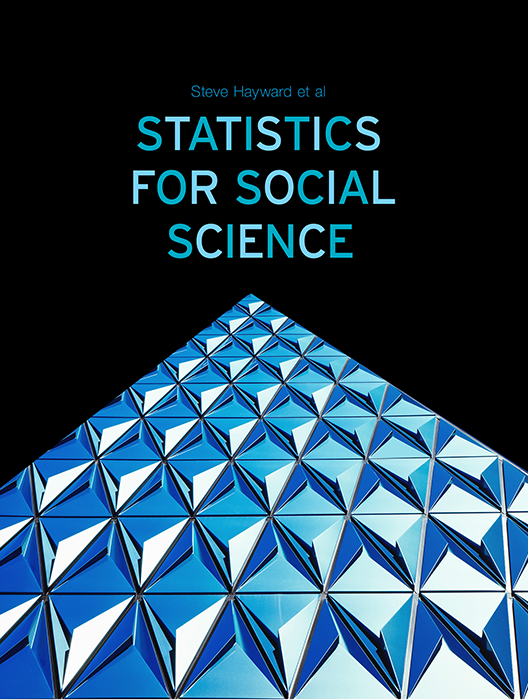Statistics for Social Science

Lead Author(s): Stephen Hayward

Statistics for Social Science takes a fresh approach to the introductory class. With learning check questions, embedded videos and interactive simulations, students engage in active learning as they read. An emphasis on real-world and academic applications help ground the concepts presented. Designed for students taking an introductory statistics course in psychology, sociology or any other social science discipline.

This content has been used by 8,525 students

What is a Top Hat Textbook?

Top Hat has reimagined the textbook – one that is designed to improve student readership through interactivity, is updated by a community of collaborating professors with the newest information, and accessed online from anywhere, at anytime.

• Top Hat Textbooks are built full of embedded videos, interactive timelines, charts, graphs, and video lessons from the authors themselves
• High-quality and affordable, at a significant fraction in cost vs traditional publisher textbooks

Key features in this textbookOur Statistics for Social Science textbook allows students to manipulate data, visualize the effects discussed, and explore Lightboard videos that feature instructor explanations to reinforce concepts and calculations.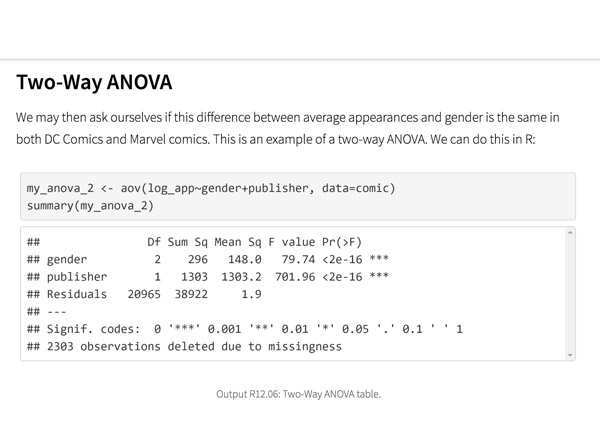Top Hat’s interactive offering includes a complementary module on using R software for data management, graphics, and conducting statistical analyses with examples and practice questions.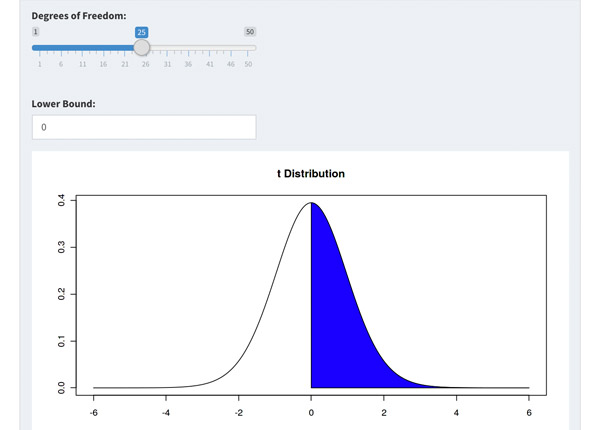Built-in assessment questions embedded throughout chapters so students can read a little, do a little, and test themselves to see what they know!

Comparison of Social Sciences Textbooks

Consider adding Top Hat’s Statistics for Social Sciences textbook to your upcoming course. We’ve put together a textbook comparison to make it easy for you in your upcoming evaluation.

Top Hat

Steve Hayward et al., Statistics for Social Sciences, Only one edition needed

Pearson

Agresti, Statistical Methods for the Social Sciences, 5th Edition

Cengage

Gravetter et al., Essentials of Statistics for The Behavioral Sciences, 9th Edition

Sage

Gregory Privitera, Essentials Statistics for the Behavioral Sciences, 2nd Edition

Pricing

Average price of textbook across most common format

Up to40-60%more affordable

Lifetime access on any device

$200.83 Hardcover print text only $239.95

Hardcover print text only

$92 Hardcover print text only Always up-to-date content, constantly revised by community of professors Content meets standard for Introduction to Anatomy & Physiology course, and is updated with the latest content In-Book Interactivity Includes embedded multi-media files and integrated software to enhance visual presentation of concepts directly in textbook Only available with supplementary resources at additional cost Only available with supplementary resources at additional cost Only available with supplementary resources at additional cost Customizable Ability to revise, adjust and adapt content to meet needs of course and instructor All-in-one Platform Access to additional questions, test banks, and slides available within one platform Pricing Average price of textbook across most common format Top Hat Steve Hayward et al., Statistics for Social Sciences, Only one edition needed Up to40-60%more affordable Lifetime access on any device Pearson Agresti, Statistical Methods for the Social Sciences, 5th Edition $200.83

Hardcover print text only

Pearson

Gravetter et al., Essentials of Statistics for The Behavioral Sciences, 9th Edition

$239.95 Hardcover print text only Sage McConnell, Brue, Flynn, Principles of Microeconomics, 7th Edition $92

Hardcover print text only

Always up-to-date content, constantly revised by community of professors

Constantly revised and updated by a community of professors with the latest content

Top Hat

Steve Hayward et al., Statistics for Social Sciences, Only one edition needed

Pearson

Agresti, Statistical Methods for the Social Sciences, 5th Edition

Pearson

Gravetter et al., Essentials of Statistics for The Behavioral Sciences, 9th Edition

Sage

Gregory Privitera, Essentials Statistics for the Behavioral Sciences, 2nd Edition

In-book Interactivity

Includes embedded multi-media files and integrated software to enhance visual presentation of concepts directly in textbook

Top Hat

Steve Hayward et al., Statistics for Social Sciences, Only one edition needed

Pearson

Agresti, Statistical Methods for the Social Sciences, 5th Edition

Pearson

Gravetter et al., Essentials of Statistics for The Behavioral Sciences, 9th Edition

Sage

Gregory Privitera, Essentials Statistics for the Behavioral Sciences, 2nd Edition

Customizable

Ability to revise, adjust and adapt content to meet needs of course and instructor

Top Hat

Steve Hayward et al., Statistics for Social Sciences, Only one edition needed

Pearson

Agresti, Statistical Methods for the Social Sciences, 5th Edition

Pearson

Gravetter et al., Essentials of Statistics for The Behavioral Sciences, 9th Edition

Sage

Gregory Privitera, Essentials Statistics for the Behavioral Sciences, 2nd Edition

All-in-one Platform

Access to additional questions, test banks, and slides available within one platform

Top Hat

Steve Hayward et al., Statistics for Social Sciences, Only one edition needed

Pearson

Agresti, Statistical Methods for the Social Sciences, 5th Edition

Pearson

Gravetter et al., Essentials of Statistics for The Behavioral Sciences, 9th Edition

Sage

Gregory Privitera, Essentials Statistics for the Behavioral Sciences, 2nd Edition

Steve HaywardRio Salado College

A lifelong learner, Steve focused on statistics and research methodology during his graduate training at the University of New Mexico. He later founded and served as CEO of Center for Performance Technology, providing instructional design and training development support to larger client organizations throughout the United States. Steve is presently lead faculty member for statistics at Rio Salado College in Tempe, Arizona.

Joseph F. Crivello, PhDUniversity of Connecticut

Joseph Crivello has taught Anatomy & Physiology for over 34 years, and is currently a Teaching Fellow and Premedical Advisor of the HMMI/Hemsley Summer Teaching Institute.

Explore this textbook

Read the fully unlocked textbook below, and if you’re interested in learning more, get in touch to see how you can use this textbook in your course today.

Tests for Goodness of Fit and IndependenceThis chapter is all about testing how changing one variable can affect another. Businesses can use these statistics in order to determine the effectiveness of new marketing campaigns and see the effect they have on their sales ​

Chapter Objectives

After completing this chapter, you will be able to:

• Determine the appropriate use of the chi-square statistic
• Identify the family of chi-square distributions as defined by their degrees of freedom
• Identify critical values of chi-square using a table
• Calculate and use chi-square in hypothesis testing
• Test whether a sample proportion is different from a population proportion
• Test whether two categorical variables are related to one another

When Would I Use Chi-Square?

Chi-square tests are used to test relationships between categorical variables at the nominal and ordinal levels of measurement. They are used for testing information about proportions or percentages. Our focus could be on the proportion of college students who live on campus or the percentage of women of childbearing age who work full-time. I.e., chi-square tests are used to test information about the proportion or percentage of people or things who fit into a category. We will consider two main types of chi-square tests here:

• Goodness of fit tests focus on one categorical variable.
• Tests of independence focus on the relationship between two categorical variables.

13.01

Chi-square tests are used for analyzing information about variables at what two levels of measurement?

13.02

Which of the following types of sample statistics are the focus of chi-square tests? (Select all that apply)

A

Means

B

Variances

C

Proportions

D

Standard deviations

E

Percentages

Goodness of Fit Test

We use the chi-square goodness of fit test to see if a proportion in a categorical variable in our sample is significantly different from the same type of proportion of interest—usually the proportion in a population.

• Does our sample proportion match or not match that of the population?
• Is our sample proportion representative of the population proportion?
• Is our sample similar to or different from the population in terms of this proportion of interest?

The goodness of fit test can be used to evaluate the representativeness of our sample. How well does our sample represent the relevant characteristics of the population?

In a goodness of fit test, we are comparing levels of one variable within a population.

Test of Independence

The test of independence is used to examine whether or not there is a relationship between two nominal and/or ordinal measures.

• Are the two variables independent (not related) or is one dependent on the other (related)?
• Is there an association or no association between them?

In a test of independence, we are comparing levels of two variables within a population.

13.03

If you were interested in testing if there is a relationship between race/ethnicity and owning vs. renting one's home would you use a chi-square test of goodness of fit or a test of independence?

A

Goodness of Fit

B

Test of Independence

13.04

If a group of researchers wishes to see if opinions about abortion in Vermont are the same as those in the U.S. as a whole, would they use a chi-square test of goodness of fit or a test of independence?

A

Goodness of Fit

B

Test of Independence

Examples: Nominal Applications

Example 1: Goodness of Fit

A social scientist was hired to select a sample of U.S. households for a survey on drug use. After the sample is selected, she compares the sample data to the most recent U.S. census data using a goodness of fit test to determine how well her sample represents the population of households in the U.S. She compares the proportions based on gender, marital status, and race/ethnicity to ensure that her sample is truly representative of the population she intends to make inferences about.

Example 2: Test of Independence

When she is satisfied with the representativeness of her sample, she begins analyzing the sample data. Now she turns her attention to tests of independence. First, she tests to see if there is a relationship between gender (male, female) and binge drinking (past month binge drinking, no binge drinking in the past month). Next, she tests for a relationship between marital status (currently married, never married, previously married) and ever having tried cocaine (tried it, did not try it).

Examples: Ordinal Applications

Example 1: Goodness of Fit

The dean of the College of Arts and Sciences is concerned about the level of student attrition, the number of students who drop out of college. He asks the faculty of the sociology department to select a sample of students and develop a survey. Responses to surveys can be biased, so he also asks the faculty to test the representativeness of the sample that responds to the survey using a goodness of fit test.

The faculty choose two key questions from the survey and ask the rest of the arts and sciences faculty to administer them to all of their majors. The questions measure students’ satisfaction with the choice of courses in the college (satisfied, unsatisfied) and the likelihood that students will leave school (quit or transfer) within the next year (extremely likely, likely, equally likely and unlikely, unlikely, extremely unlikely). They then compare the proportions of responses to these two survey questions in the online sample to the population of students to see if their sample is significantly different from the population.

Example 2: Test of Independence

Once satisfied with the representativeness of the sample of students in the College of Arts and Sciences, the dean meets with the sociology faculty to discuss analysis plans. The dean was especially interested in learning at what point in their time at the university do students start thinking about quitting or transferring.

The faculty test to see if there is a relationship between year in school (first, second, third, fourth, fifth, more than fifth) and the probability of leaving school, and between satisfaction with course offerings and the probability of quitting or transferring in the coming year. In other words, is the decision to leave school related or not related to year in school and/or college course offerings?

The Chi-Square Distribution

Degrees of Freedom

Chi-square is really a family of probability distributions. Their individual shapes depend on their respective degrees of freedom (df). You will recall from previous lessons that degrees of freedom refer to the number of values in a calculated statistic that are free to vary (do not have a fixed value). For example, if you are given the first three numbers in a distribution of four numbers as 2, 4, and 5, and then must identify the fourth number so that all four numbers will add to 20, the fourth number must be 9. The first three numbers can vary but once they are determined, the last number is no longer free to vary. So we say that this distribution has n – 1 degrees of freedom, or df = 3.

Family of Distributions

The shapes of the distributions look like this:Figure 13.1:​ Family of shapes for the chi-square distribution

Do you see the family resemblance? The x-axis (horizontal) of this graph presents the values of chi-square (χ2= 0 to 50), and the y-axis (vertical) presents the probability (p = .00 to .30) of these values. With only a few degrees of freedom, the shape of the distribution is severely skewed to the right, i.e., it is positively skewed—it has a long skinny tail on the right side of the distribution. As the degrees of freedom (which are directly related to the number of categories of the variable or variables, as we will see shortly) increase, the shape of the distribution approaches normal and becomes more bell-shaped and symmetrical. With as many as 15 degrees of freedom, the shape of the probability distribution is getting close to normal.

13.05

Which one of the following best describes the chi-square distribution?

A

It is normal in shape.

B

It is skewed to the left.

C

It is a family of shapes dependent on sample size.

D

It is a family of shapes dependent on the degrees of freedom.

13.06

The degrees of freedom for chi-square depend on what?

A

Sample size

B

Number of statistics

C

Number of categories or levels in the variables

D

Number of variables in the dataset

Adjust the Degrees of Freedom slider to see the effect of changing df on the shape of the chi-square distribution and the rejection region.This Content is Locked
Only a limited preview of this text is available. You'll need to sign up to Top Hat, and be a verified professor to have full access to view and teach with the content.

Assumptions of the Chi-Square Test

Although the chi-square probability distributions approach normal in shape as the degrees of freedom increase, there is no need to assume the original distribution of the population is normal. There is no requirement concerning the shape of the population distribution to use chi-square. In fact, the only requirements to use chi-square generally are that:

• The sample was chosen using a random sampling method.
• The variables being analyzed are categorical (nominal or ordinal).
• An additional requirement dependent on whether it is a test of goodness of fit or a test of independence, which we will discuss in their respective sections below.

13.07

Which of the following is not a necessary assumption to use the chi-square distribution?

A

The sample distribution is normal in shape.

B

The variables to be analyzed are categorical.

C

The sample was selected using a random method.

Critical Values for Rejecting the Null Hypothesis

Partly because the probability distribution of chi-square is positively skewed, we will focus only on the upper tail to determine whether or not we can reject the null hypothesis using chi-square. We don’t have to be troubled with the question of lower tail or two-tail tests. With chi-square, it is always an upper-tail, or right-tailed, test, as illustrated in this graph: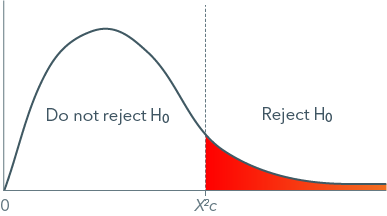Figure 13.2:​ Rejection region for the chi-square distribution

The graph above shows the rejection region, the area to the right of the critical value of chi-square, to which we compare our calculated chi-square value. If our calculated value is greater than or equal to the critical value, it falls into the rejection region and we can reject the null hypothesis of equality (for goodness of fit) or independence (for tests of independence). In that case, there is support for the alternative hypothesis. If our calculated chi-square is less than the critical value, we must fail to reject the null hypothesis, and the alternative (research) hypothesis is not supported. You will recall that we never, ever, at any point in time, under any circumstances, “accept” the alternative hypothesis. The way in which we determine the critical value of chi-square and calculate the chi-square for our study is discussed shortly.

13.08

How many tails of the chi-square distribution are used to test hypotheses?

13.09

Match the following decisions with the comparison of calculated and critical chi-square values.

Premise
Response
1

The calculated chi-square is greater than the critical chi-square

A

Fail to reject the null hypothesis

2

The calculated chi-square is less than the critical chi-square

B

Fail to reject the null hypothesis

3

The calculated chi-square is equal to the critical chi-square

C

Reject the null hypothesis

D

Reject the null hypothesis

13.10

In the below chi-square distribution, the critical value is 5.99. Click on the hotspot in the appropriate area of the distribution if a chi-square of 8.34 is calculated.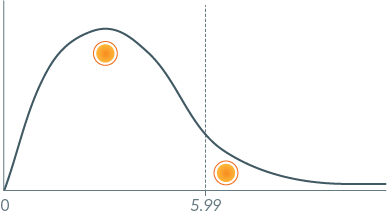13.11

In the below chi-square distribution, the critical value is 2.71. Click on the hotspot in the appropriate area of the distribution if a chi-square of 2.45 is calculated.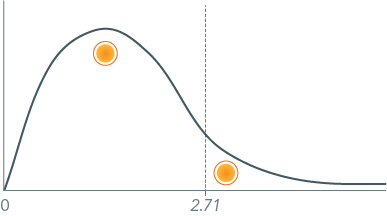Examples: Tests Where the Null Hypothesis is Rejected

Example 1: Goodness of Fit

A political scientist is interested in polling the votes in the last presidential election. She designs an exit poll for all voting districts in Elmira, NY. After collecting the data, she is curious to see if the political affiliation (Republican, Independent, Democratic) of actual voters is representative of the affiliation of all registered voters in Elmira. This is a goodness of fit test. She determines the critical value of chi-square that is appropriate for her study (details of this are discussed below). The value is 5.99. She then calculates chi-square and gets 101.44. She rejects the null hypothesis and concludes that the political affiliation of actual voters is not representative of the affiliation of all registered voters in Elmira, NY. Her sample is biased in terms of not representing the political party proportions of registered voters in Elmira. However, this could be an interesting result, too—people of certain political affiliations may be more or less likely to get out and vote.Figure 13.3:​ Specific rejection region for a chi-square critical value of 5.99

Example 2: Test of Independence

The social scientist working on the survey of drug use discussed earlier tests the relationship between marital status and ever having tried cocaine. The critical value for this test of independence is 9.21. Her calculated chi-square is 12.95. She rejects the null hypothesis of independence and concludes that marital status and cocaine experimentation or use are associated in some way.

Examples: Tests Where the Null Hypothesis is Not Rejected

Example 1: Goodness of Fit

A social scientist is working on the household survey of drug use tests to see if her household sample is representative of the gender distribution in the U.S. population. The critical value of chi-square for this goodness of fit test is 6.63. Her calculated chi-square is 0.23. She cannot reject the null hypothesis, which in this case is a desirable result. The distribution of gender in her sample is not different from that in the population. Her sample is representative when it comes to gender.Figure 13.4:​ Specific Rejection Region for a Chi-Square Critical Value of 6.63

Example 2: Test of Independence

The dean of the College of Arts and Sciences discussed earlier asks the sociology faculty to test the relationship between students’ satisfaction with the college’s course offerings and whether they are likely to leave college in the coming year. The critical value of chi-square for this test of independence is 9.49. Their calculated chi-square is 0.08. They cannot reject the null hypothesis of independence, and conclude that course offerings are not related to leaving school in the coming year. The two variables are independent. His students are leaving school for some other reason.

The Chi-Square Statistic

The general purpose of chi-square is to compare frequencies and determine whether or not the differences are large enough to reject the null hypothesis. Are they statistically significant? Most simply, the chi-square statistic is the sum of proportional differences between observed and expected frequencies. The expected frequencies are hypothetical. They are the frequencies we would expect if the null hypothesis is in fact a true statement. If the actual or observed frequencies are different enough from the expected, the null hypothesis can be rejected. Sample proportions are not equal to population proportions (goodness of fit test) or two variables are not independent (test of independence).

Expected and Observed Frequencies

The chi-square statistic is used to compare expected frequencies to observed frequencies in categorical variables.

• In a goodness of fit test, it compares the frequencies we would expect if our sample proportions are equal to the actual or hypothesized population proportions.
• In a test of independence, it compares the frequencies actually observed to the frequencies we would expect if two variables are not associated in some way, i.e., if they are independent.

Hence, before we can calculate the chi-square statistic, we must calculate the expected frequencies for the purposes of comparison. The formula for calculating expected frequencies differs for goodness of fit and independence tests. These expected frequency formulae are covered in their respective sections below.

The Chi-Square Formula

For now, we will examine the formula for chi-square, which is the same for both types of tests. We will discuss each component, define the notation, and calculate several examples. The formula is:

The formula uses O as the observed frequency and E as the expected frequency. Each squared difference for each category of the variable divided by the corresponding expected frequency is then summed to produce the chi-square value. The differences are squared so that the resulting chi-square will equal a value other than zero. The formula compares the squared differences between the observed and expected frequencies as a proportion of the expected frequency.

The table below summarizes the order of operations for this formula.Figure 13.5:​ Order of Operations for Calculating the Chi-Square Statistic
13.12

A researcher calculating a chi-square statistic determines that observed frequencies total 5104 while expected frequencies total 5080. What is the chi-square value to the nearest hundredth?

Limitations of the Chi-Square Statistic

As with most statistics, chi-square has its limitations.

• First, the value of chi-square tells us nothing about how much different the sample is from the population or how strong the relationship is between the two variables. In addition, for the latter test, the statistic also tells us nothing about the direction of the relationship. We must visually compare the frequencies (or percentages) to get some idea about the amount of difference and the direction and strength of a relationship.
• Second, the statistic is sensitive to sample size. The larger the sample, the larger the value of chi-square, independent of the amount of difference or strength of the relationship.
• Third, chi-square is unstable if the frequencies (observed and expected) are small in one or more categories of the variable or cells in the table. A frequency is considered small if it is less than 5.

13.13

Sort these operations for calculating chi-square into the correct order.

A

Sum all the quotients.

B

Subtract the expected frequency from the observed frequency.

C

Square the difference.

D

Divide the squared difference by the expected frequency.

13.14

What are some of the limitations of the chi-square test statistic? (Select all that apply)

A

Chi-square is influenced by sample size.

B

Chi-square tells nothing about the strength of a relationship between two variables.

C

Chi-square tells nothing about the direction of a relationship between two variables.

D

Chi-square can only be used with continuous variables.

E

Chi-squares tells nothing about statistical significance.

F

Chi-square is limited to sample distributions that are normal in shape.

13.15

The larger the sample size, the _________ the chi-square value.

13.16

Generally, what is the smallest size in a variable category or table cell that chi-square can tolerate?

Examples: Chi-Square Calculations

Example 1: Goodness of Fit

The political scientist examining the voting behavior in Elmira, NY, observed a total (n) of 9,306 voters. She observed 6,051 Republicans but the number that would be expected according to voter registration is 5,583.6. She observed 864 Independents but expected 930.6. She observed 2,391 Democrats but expected 2791.8. The calculation is as follows:

Note how large this chi-square value is, due in part to the large sample size of 9,306. The other reason for the value to be so large is the large differences between the observed and expected frequencies. This value is certainly large enough to reject the null hypothesis and conclude that the social scientist’s sample voters are not representative of the population of registered voters in Elmira.

Example 2: Test of Independence

The dean’s sample included 249 students. With data from the entire college, the sociology faculty calculated a chi-square of 0.08 in a test of independence between satisfaction with course offerings and the likelihood of leaving school in the coming year. They observed the following: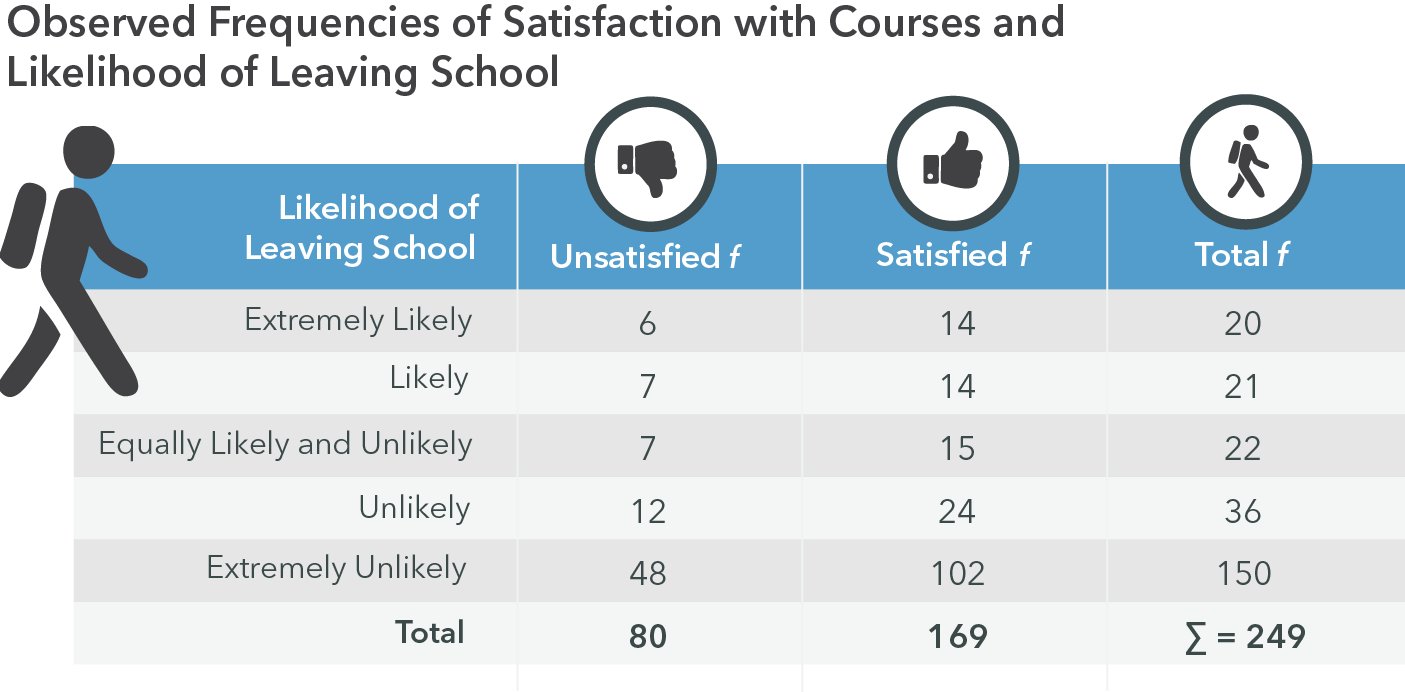Figure 13.6:​ Observed frequencies of satisfaction with courses and likelihood of leaving school

The frequencies in this table are what they observed. The expected values, assuming independence between the variables are: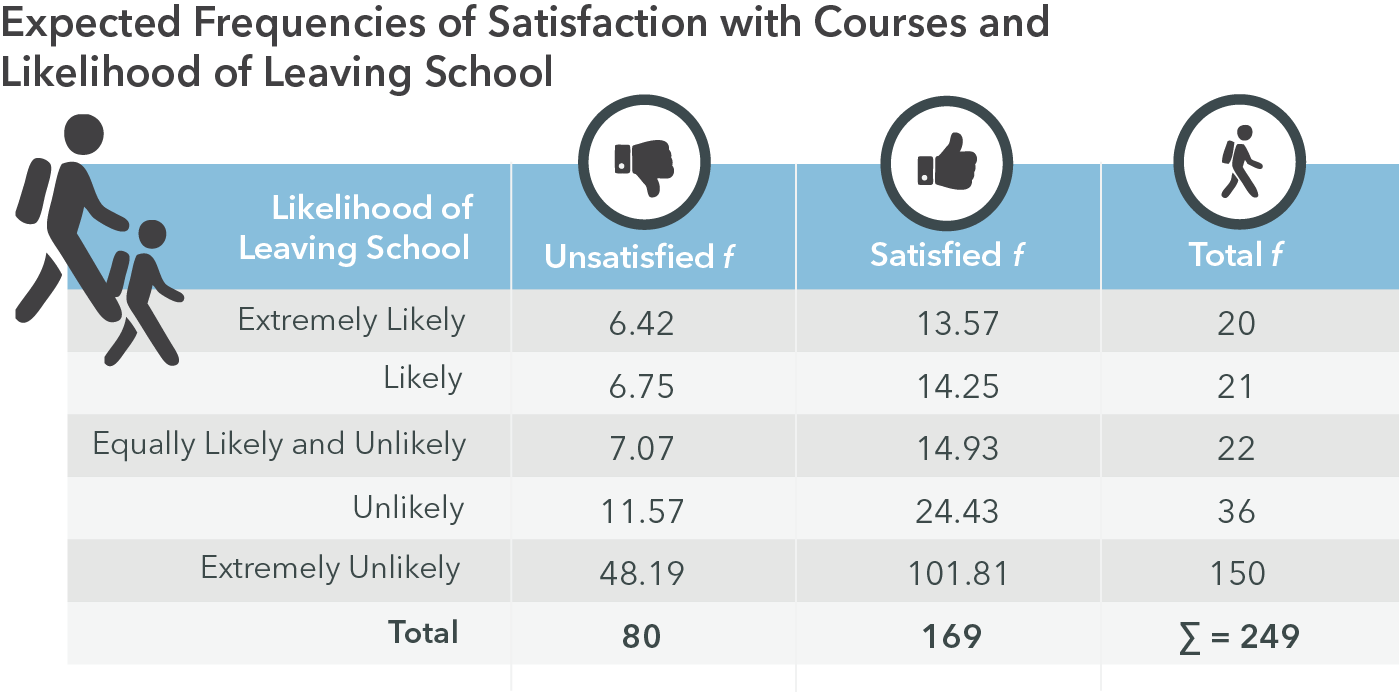Figure 13.7:​ Expected frequencies of satisfaction with courses and likelihood of leaving school

The calculation of chi-square for this test of independence is as follows:

Such a small chi-square value suggests that the null hypothesis cannot be rejected. Satisfaction with course offerings and likelihood of leaving school are independent. They are not related.

Using the Chi-Square Table

Most versions of the chi-square table present the critical values of chi-square associated with various degrees of freedom and alpha levels. They can go on for pages and pages. A relatively simple example of the table looks like this: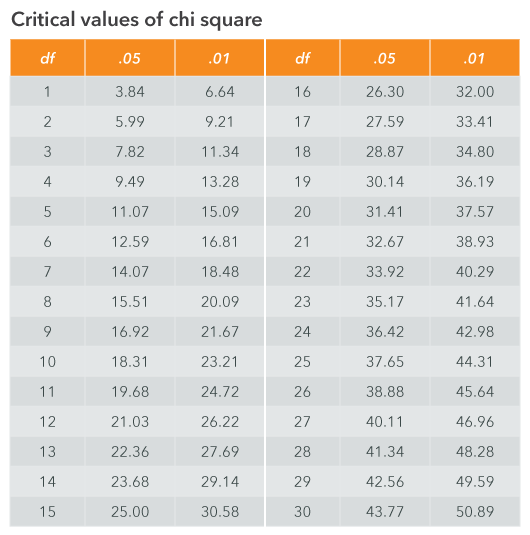Figure 13.8:​ Critical values of chi-square​​

Explanation of the Table

The columns indicate two levels of alpha, .05 and .01. Other versions of this table can be quite comprehensive and include several alphas or probabilities of chi-square and many more rows of degrees of freedom. The table above is sufficient for most chi-square tests. Few chi-square tests involve even as many as 30 degrees of freedom since the degrees of freedom are tied to the number of categories of the categorical variables being analyzed. It’s somewhat uncommon to analyze variables with more than five or six categories in the social sciences. So a table with 30 degrees of freedom is adequate in most cases. (And you can fit the table on one page!)

The alpha levels indicate the level of significance for which the null hypothesis will be rejected or not rejected. The most commonly chosen alpha is .05; an alpha of .01 is a more conservative or demanding test because of its smaller tail in the chi-square distribution. Other alpha levels that are often used are .10 and .001. A more complete table would include these alpha levels, but this table will serve our purposes in learning about chi-square. The use of the table simply involves finding the chi-square value associated with the appropriate number of degrees of freedom (the rows) and the chosen alpha level (the columns).

Example: Using the Chi-Square Table

The political scientist conducting the political polling study in Elmira, NY, determined that the critical value of her study is 5.99. She chose an alpha level of .05 and has 2 degrees of freedom. (We will discuss the ways to calculate degrees of freedom shortly.) Using the chi-square table, she looks in the row for 2 degrees of freedom and column for .05. The value in that row and column is 5.99.

The political scientist decides to analyze voting behavior by neighborhood of residence in Elmira, NY. The U.S. census classifies 42 neighborhoods in Elmira. In this example, she has more than 30 degrees of freedom, but the table only has enough rows for 30 degrees of freedom. The political scientist knows it’s acceptable to use the critical value for 30 degrees of freedom and her choice of alpha (.01 in this case). She finds the critical value of chi-square in the 30 degrees of freedom row and the .01 column to be 50.89. This strategy is appropriate with degrees of freedom that are larger than the numbers presented in any version of the chi-square table.

13.17

Click on the hotspot next to the critical value of chi-square with an alpha of .05 and 18 degrees of freedom.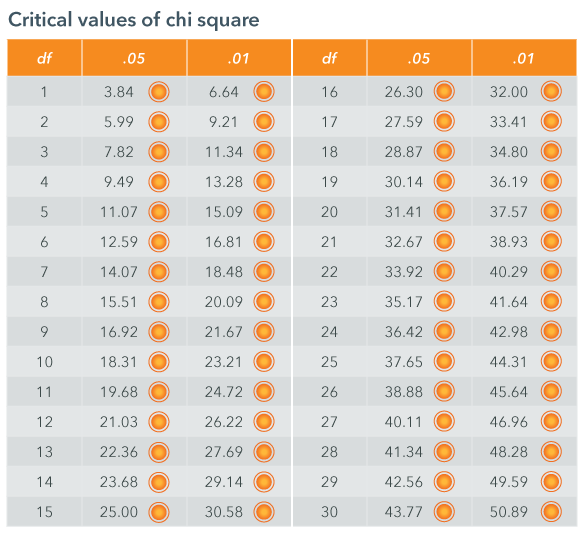13.18

Click on the hotspot next to the critical value of chi-square with an alpha of .01 and 5 degrees of freedom.13.19

Click on the hotspot next to the critical value of chi-square with an alpha of .05 and 29 degrees of freedom.Chi-Square Tests Overview

Now we’re ready to put all of this together in order to conduct these tests using chi-square. As a review, the steps of hypothesis testing are summarized in the table below.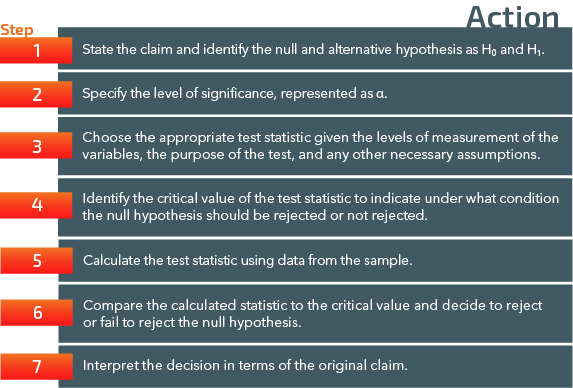Figure 13.9:​ The steps of hypothesis testing

We will follow these steps for both types of chi-square tests—goodness of fit and independence.

The specific null and research hypotheses, the formulae for determining the degrees of freedom, and the method for calculating the expected values are different for the two types of tests. The use of the chi-square table and the formula for calculating chi-square are the same for both tests.

Question 13.20

13.20

In hypothesis testing, what is the purpose of choosing an alpha level?

Question 13.21

13.21

What is the purpose of the decision rule?

Question 13.22

13.22

What decision is made in Step 6 of the hypothesis test where the calculated statistic is compared with the critical value?

Goodness of Fit Test

In a goodness of fit test we are comparing levels of one variable within a population.

Sometimes we want a good fit (i.e., hoping our sample is representative), and sometimes we don’t (i.e., a difference is a meaningful research result). Examples of relevant research questions are:

• Is the religious affiliation of a random sample of residents in Oak Park, IL representative of the religious affiliation of Illinois residents as a whole?
• Is the percentage of unemployed immigrants in Paris, France representative of the percentage of immigrants in the entire Ile-de-France region?
• Is the proportion of youth who are obese in Sacramento, CA in 2015 different from the proportion in 1995?
• Has the proportion of African American men in federal prisons changed from that prior to the “war on drugs” in the 1980s?
13.23

What does chi-square goodness of fit actually test?

A

If sample means are equal to or not equal to population means

B

If two continuous variables are independent or not

C

If two categorical variables are independent or not

D

If sample proportions are equal to or not equal to population proportions

Method and Interpretation for the Goodness of Fit Test

The formula for chi-square in the goodness of fit test is:

Expected Frequencies for the Goodness of Fit Test

Recall that O is the observed frequency and E is the expected frequency. The general idea is to apply the proportions in the population or other comparison group to our particular sample. If the proportion of interest in the population is .75, then we would calculate the expected frequency for a proportion of .75 in our sample as .75 x sample size n. So, the general formula for expected frequencies in the goodness of fit test is:

These are the expected values if the proportions in our sample are the same as those in the group to which we’re comparing.

13.24

With a sample size of 1000 and a proportion of .86, what is the expected frequency for the chi-square calculation?

13.25

What exactly does the expected frequency for the goodness of fit test indicate?

A

The cell frequency if two categorical variables are independent.

B

The category frequency if the population proportion is equal to the sample proportion.

C

The cell frequency if two continuous variables are independent.

D

The category frequency if the population proportion is not equal to the sample proportion.

Examples of Expected Values for Goodness of Fit Tests

The dean of the College of Arts and Sciences has a sample of 249 students. He wishes to test whether or not the sample is representative of the likelihood of leaving school for all students in the college. The proportions in the census study of all students in the college are: .08 who are extremely likely to leave school in the coming year, .08 who are likely, .09 who are equally likely and unlikely, .15 who are unlikely, and .60 who are extremely unlikely. Using the formula for expected values (proportions in the population multiplied by the sample size), the expected values are: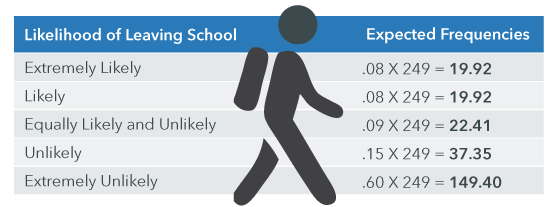Figure 13.10:​ Expected frequencies of the likelihood of leaving school​

The political scientist in Elmira, NY has a sample of 9,306 voters. She checks the representativeness of her sample by applying the proportions of political affiliation of all registered voters in Elmira to her sample size in order to calculate the expected frequencies if the proportions in her sample of voters were the same as those in the population of registered voters. The proportions of registered voters are: .60 Republican, .10 Independent, and .30 Democratic. The expected values are: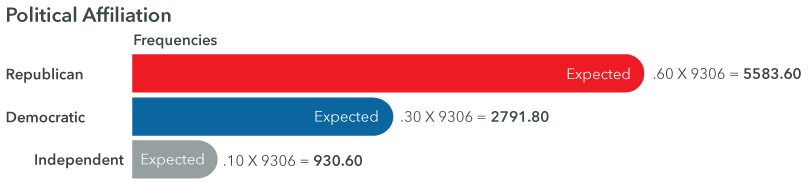Figure 13.11:​​ Expected frequencies of political affiliation​

Degrees of Freedom for the Goodness of Fit Test

The last thing to learn about the chi-square goodness of fit test is the formula for calculating the degrees of freedom. The formula for goodness of fit is:

where k is the number of categories, or levels, in the variable of interest. For the dean’s study, the degrees of freedom is (5 - 1) = 4 because the measure of likelihood of leaving school has five categories (extremely likely, likely, equally likely and unlikely, unlikely, and extremely unlikely). For the political scientist’s study, the degrees of freedom is (3 - 1) = 2 because political affiliation has three categories (Republican, Independent, and Democratic).

13.26

When calculating the degrees of freedom for chi-square, what does the variable "k" stand for?

13.27

A chi-square test using the variable social class (upper, middle, lower, and poverty) has how many degrees of freedom?

Chi-Square Goodness of Fit Hypothesis Test

We’ll go through the hypothesis testing process one step at a time.

Step 1: State the claim and identify the null and alternative hypotheses.

The null hypothesis for the goodness of fit test is that each proportion in the sample is equal to the equivalent proportion in the population. For example, if the population proportions are: p1=.200; p2=.300; p3=.250; and p4=.250, the null hypothesis can be stated as:

The alternative hypothesis can be simply stated as:

H1: the null hypothesis is false

Step 2: Specify the level of significance.

Step 3: Choose the appropriate test statistic given the levels of measurement of the variables, the purpose of the test, and any other necessary assumptions.

The chi-square goodness of fit statistic is chosen assuming the variable of interest is categorical, the sample has been chosen randomly, and each category of the variable has a frequency of at least five (5).

Step 4: Identify the critical value of the test statistic to indicate under what condition the null hypothesis should be rejected or not rejected.

Using the alpha value specified in step two and the degrees of freedom (k - 1), find the critical value in the chi-square table. State:

H0 will be rejected if calculated chi-square is greater than or equal to the critical value of chi-square.

Step 5: Calculate the test statistic using data from the sample.

Step 6: Compare the calculated statistic to the critical value to determine whether or not the null hypothesis should be rejected.

Step 7: Interpret the results in words.

Reject the null hypothesis if the calculated chi-square is greater than or equal to the critical value. Fail to reject the null hypothesis if the calculated chi-square is less than the critical value.

13.28

For the goodness of fit test, the null hypothesis generally states which of the following?

A

The alternative hypothesis is true

B

Chi-square equals zero (0)

C

The population and sample proportions are equal

D

The population and sample proportions are not equal

13.29

For the goodness of fit test, the alternative hypothesis generally states which of the following?

A

The alternative hypothesis is false

B

The null hypothesis is true

C

Chi-square does not equal zero (0)

D

The population and sample proportions are not equal

13.30

If the calculated chi-square is greater than the critical value, what is the conclusion?

A

We fail to reject the null hypothesis

B

We accept the alternative hypothesis

C

We reject the null hypothesis

D

We reject the alternative hypothesis

13.31

If the null hypothesis is rejected, would we conclude that the population and sample proportions are equal or unequal?

Examples of Chi-Square Goodness of Fit Tests

We return to the political scientist studying voting behavior in Elmira, NY. By examining the city voting registration records, she determines that in the population of Elmira, 60% (p1) of registered voters are Republican, 10% (p2) are Independent, and 30% (p3) are Democratic. She uses these proportions to calculate the expected values for her sample of voters (n=9306).Figure 13.12:​​ Expected frequencies of political affiliation​

She chooses an alpha level of .05. She is now ready to test her hypotheses using the five steps for the test.

Step 1: H0: p1 = .30; p2 = .10, p3 = .30
H1: H0 is false

Step 2: α = .05

Step 3:

Step 4: The degrees of freedom are (3 - 1) or 2; and the alpha is .05. The critical value of chi-square is 5.99. Reject H0 if χ2≥ 5.99

Step 5: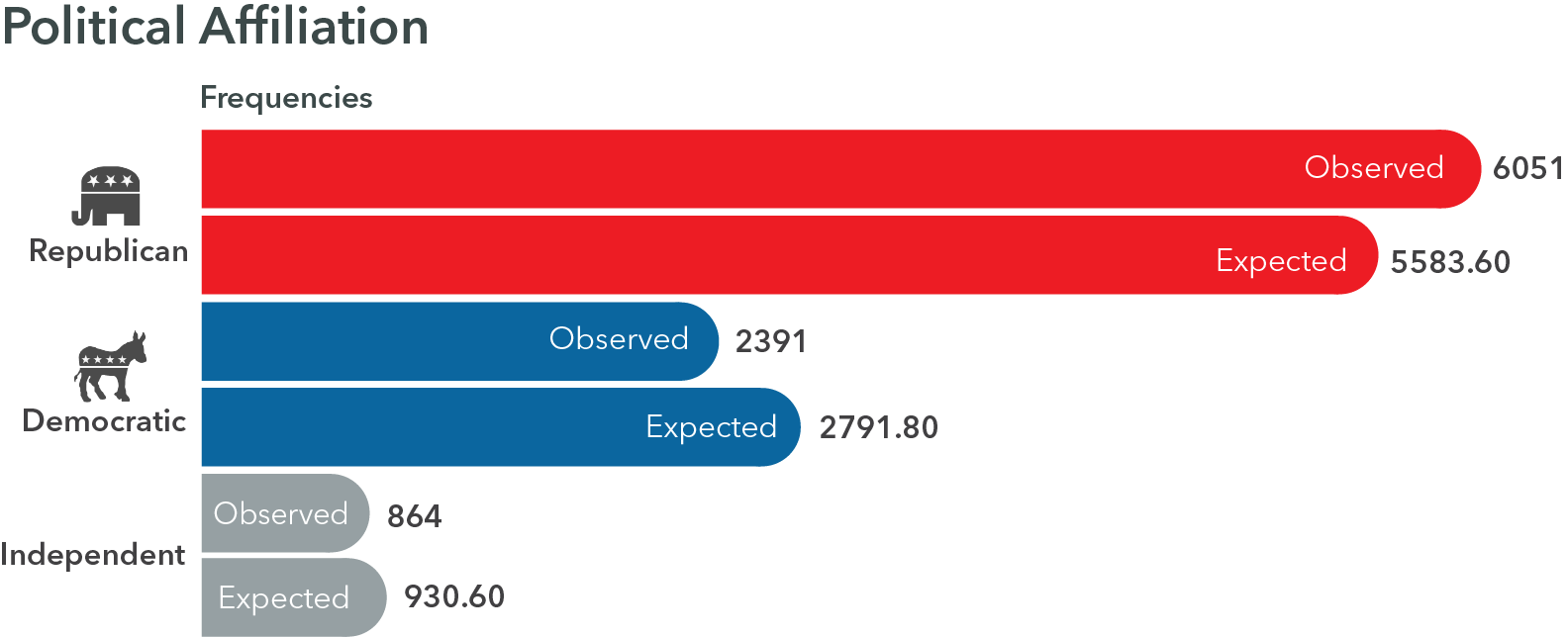Figure 13.13:​​ Observed and expected frequencies of political affiliation

Step 6: Reject H0 because 101.44 > 5.99.

Step 7: We have evidence at an alpha of .05, that the null hypothesis (the sample and population proportions are equal) is false. The sample proportions are not equal to the population proportions.

The political scientist’s sample of voters in Elmira, NY is not representative of the population of registered voters in terms of political affiliation. For her purposes, this is a meaningful result. Folks who actually vote are rarely if ever representative of the population of registered voters.

Back to the social scientist conducting the household survey on drug use. One of her tasks is to test the representativeness of her sample in terms of gender. She consults the 2010 U.S. Census data and finds that 49.2% of the population is male and 50.8% are female. The census does not have a category for other genders, so she uses the dichotomous classification. Her sample size is 10,000, so her expected values are:Figure 13.14: Expected frequencies of gender​​​

The social scientist chooses a conservative alpha of 0.01 for this test because gender representativeness is a key requirement for the household survey. She is now ready to test her hypotheses.

Step 1: H0: p1=.492; p2=.508
H1: H0 is false.

Step 2: α = .01

Step 3:

Step 4: With (2-1) or 1 degree of freedom, and alpha .01, the critical value is 6.63. Reject H0 if χ2 ≥ 6.63

Step 5: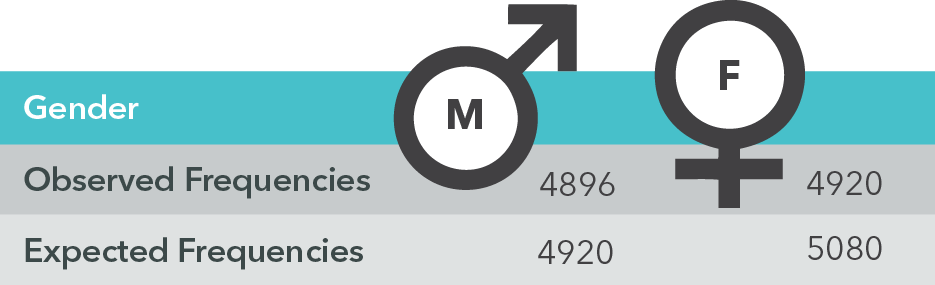Figure 13.15:​​ Observed and Expected Frequencies of Gender

Step 6: Fail to reject the H0 because 5.16 < 6.63.

Step 7: We do not have evidence at alpha .01 that the null hypothesis is not true. The proportions of the sample and population are close to equal. Her sample is representative of the population in terms of gender.

Test of Independence

In a test of independence we are comparing levels of two variables within a population. It determines whether or not there is a statistically significant relationship between two categorical variables. Variables are independent if they are not related, meaning they are not associated in any meaningful way and therefore do not have an effect on each other. The test is based on frequencies presented in contingency tables, which are tables showing the frequencies of two variables simultaneously. A contingency table looks like this: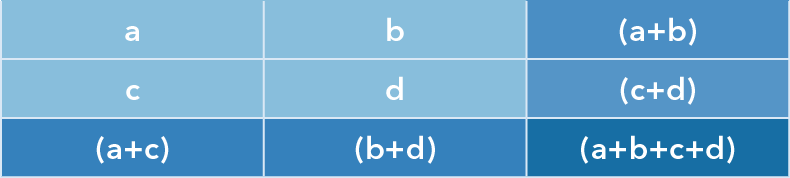Figure 13.16: ​​General example of a contingency table

The individual lower case letters represent cell frequencies. The letter “a” represents the frequency of the sample in the first category of the column variable and the first category of the row variable. The summed lower case letters represent the table marginals. Marginals are the column (a+c or b+d), row (a+b or c+d), and table (a+b+c+d) totals. The marginal values are important for calculating the expected cell frequencies, as you will see shortly. The chi-square calculation compares the observed and expected frequencies in each and every cell of the table. If chi-square is calculated by hand, you will hope that the variables do not have a lot of categories! In everyday practice, technology tools greatly simplify the work.

A more specific and extremely important contingency table:Figure 13.17:​​ Frequency distribution of hair color and fun

Do blondes really have more fun? Who knows?

13.32

What is the purpose of the chi-square test of independence?

A

To compare two or more means

B

To compare population and sample proportions

C

To determine whether or not two continuous variables are independent

D

To determine whether or not two categorical variables are independent

13.33

Column and row totals are collectively called what?

Method and Interpretation of the Chi-Square Test of Independence

We use the table marginals to calculate the expected frequencies for the chi-square test of independence. The expected values are those that we would see if the two variables are totally independent—there is no relationship between them. Chi-square compares the expected cell frequencies (assuming independence) to the observed cell frequencies to see if the observed values are different enough from the expected values to suggest a statistically significant relationship between the two variables.

Expected Frequencies for the Test of Independence

The formula for calculating the expected values for the test of independence is:

Let’s examine the calculation of expected values for each cell of the general contingency table.

For cell a, the expected frequency is:

For cell b, the expected frequency is:

For cell c, the expected frequency is:

For cell d, the expected frequency is:

For each cell of the table, these expected frequencies are subtracted from the corresponding observed frequency in the same cell. Each difference is squared and divided by the respective expected frequency, and all quotients are summed to calculate the chi-square value.

Degrees of Freedom for the Independence Test

The calculation for degrees of freedom is based on the number of categories for each variable. The formula is expressed by using information from the contingency table. How many rows are there? How many columns are there? The formula is:

where r is the number of rows and c is the number of columns.

A table with 4 rows and 5 columns has df equal to (4 - 1)(5 - 1) = (3)(4) = 12.

A table with 3 columns and 3 rows has df equal to (3 - 1)(3 - 1) = (2)(2) = 4.

13.34

What are the degrees of freedom for a chi-square test of independence for the following table?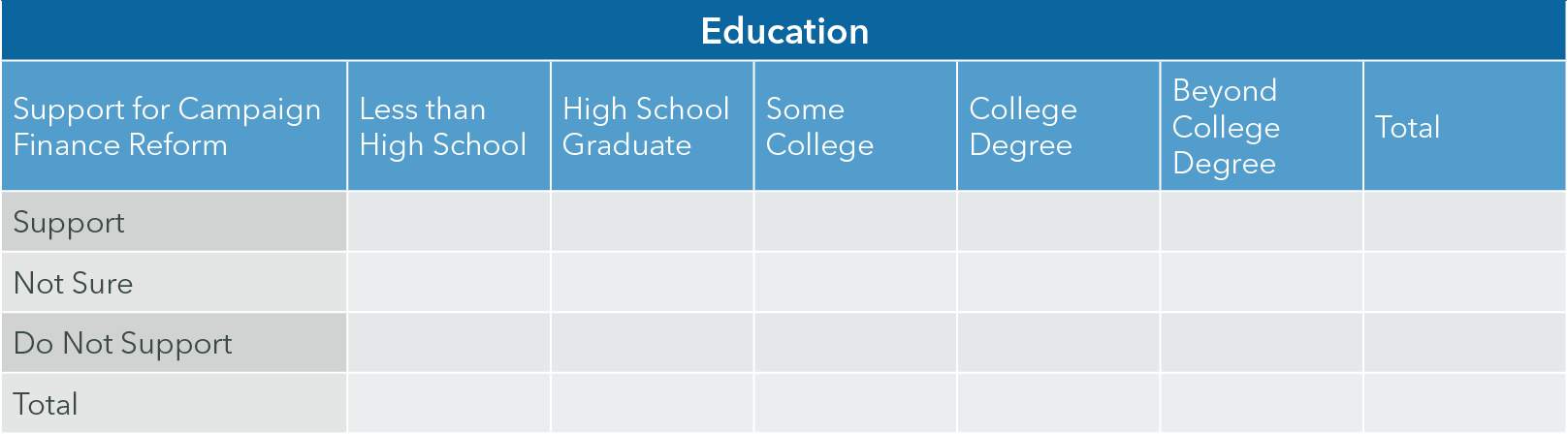13.35

One component of the chi-square calculation is $\frac{(14-13.57)^2}{13.57}$. What is the solution to the nearest hundredth?

Chi-Square Independence Hypothesis Test

Now we can examine the hypothesis testing process for the test of independence. The steps are:

Step 1: Specify the null and alternative hypotheses.

The null hypothesis states that the two variables are independent. The alternative hypothesis is that the variables are not independent, implying that there is support for a relationship between the two variables.

• H0: Variable A and variable B are independent.
• H1: Variable A and variable B are not independent.

Step 2: Specify the alpha level.

Step 3: Choose the statistic based on the necessary assumptions.

Step 4: Determine the critical value for the purpose of rejecting or not rejecting the null hypothesis.

• Calculate the degrees of freedom (r - 1)(c - 1) and use the alpha specified in step 1 to select the critical value of chi-square from the chi-square table.
• H0 is rejected if the calculated chi-square is greater than or equal to the critical value.

Step 5: Calculate the chi-square value.

Step 6: Compare the calculated chi-square value to the critical chi-square value to determine whether or not the null hypothesis should be rejected.

Step 7: Interpret the results relative to the original claim.

• Interpret the results such that the variables are independent (not related) if we fail to reject the null hypothesis. If we can reject the null hypothesis, we have support that the variables are not independent (they are related).

13.36

For the test of independence, the null hypothesis generally states which of the following?

A

The two variables are not independent

B

Chi-square equals zero (0)

C

The population and sample proportions are equal

D

The two variables are independent

13.37

For the test of independence, the alternative hypothesis generally states which of the following? (Select all that apply)

A

The alternative hypothesis is false

B

The null hypothesis is false

C

Chi-square does not equal zero

D

The two variables are not independent

E

The two variables are related to one another

13.38

If the calculated chi-square is less than the critical value, what is the conclusion?

A

We fail to reject the null hypothesis

B

We accept the alternative hypothesis

C

We reject the null hypothesis

D

We reject the alternative hypothesis

13.39

If the null hypothesis is rejected, we would conclude that the two variables are ________ .

A

not independent

B

independent

Examples of Chi-Square Tests of Independence

Returning to the dean’s sample of 249 students and the question about the relationship between college course offerings and the likelihood of leaving school in the coming year. The hypothesis test is:

Step 1: H0: Satisfaction with course offerings and the likelihood of leaving school are independent.
H1: Satisfaction with course offerings and the likelihood of leaving school are not independent.

Step 2: α = .05

Step 3:

Step 4: df = (5 - 1)(2 - 1) = 4; alpha is .05; Reject H0 if χ2 ≥ 9.49

Step 5: Calculate the expected values assuming the variables are independent.Figure 13.18:​​ Observed frequencies of satisfaction with courses and likelihood of leaving schoolFigure 13.19:​​ Expected frequencies of satisfaction with courses and likelihood of leaving schoolFigure 13.20: ​​Observed and expected frequencies of satisfaction with courses and likelihood of leaving school

Step 6: Fail to reject the null hypothesis because 0.08 is not greater than or equal to 9.49.

Step 7: Satisfaction with courses and likelihood of leaving school are independent.

Case Study: Sleep Patterns among Adults in Different Family Types

The following case study will allow us to apply the chi-square test of independence to a real-world problem. According to a recent research article comparing sleep patterns among adults in different family types, single parents get less sleep than both two-parent families and adults without children. The authors conclude that these differences are statistically significant, but specific results of their hypotheses tests are not included in the original article. They analyzed data from the 2013-2014 National Health Interview Survey with a sample size of about 35,000 adults.

The results showed that 31.0% of adults living without children, 42.6% of single parents, and 32.7% of adults in two-parent families got less than 7 hours of sleep daily.

And what is the ideal amount of sleep per night? Watch below to learn more.

Case Study Question 13.01

Case Study Question 13.01

Convert these percentages to observed frequencies using a pen and paper. Assume that 10,000 adults are childless, 10,000 are single parents, and 15,000 are adults in two-parent families.

Click here to see the answer to Case Study Question 13.01

Case Study Question 13.02

Case Study Question 13.02

Calculate the expected frequencies using a pen and paper.

Click here to see the answer to Case Study Question 13.02.

Case Study Question 13.03

Case Study Question 13.03

Conduct the appropriate hypothesis test. What is the correct decision?

A

Reject the null hypothesis

B

Fail to reject the null hypothesis

Click here to see the answer to Case Study Question 13.03.

Case Study Question 13.04

Case Study Question 13.04

Are these differences really statistically significant (alpha of 0.05)?

Click here to see the answer to Case Study Question 13.04.

Case Study Question 13.05

Case Study Discussion 13.05

How might sample size influence the results of the hypothesis test?

Click here to see the answer to Case Study Discussion 13.05.

References

Nugent CN, Black LI. Sleep duration, quality of sleep, and use of sleep medication, by sex and family type, 2013–2014. NCHS data brief, no 230. Hyattsville, MD: National Center for Health Statistics. 2016. Retrieved from: http://www.cdc.gov/nchs/data/databriefs/db230.pdf

Nierenberg, C. (2016, January 6). Missing Zzzs: Sleep Problems Common for Single Parents, Women. Retrieved from LiveScience: http://www.livescience.com/53281-sleep-problems-common-single-parents-women.html

Pre-Class Discussion Questions

Class Discussion 13.01

Class Discussion 13.01

Create a research question for which it would be appropriate to use the chi-square goodness of fit test. State the null and alternative hypotheses.

Click here to see the answer to Class Discussion 13.01.

Class Discussion 13.02

Class Discussion 13.02

Create a research question for which it would be appropriate to use the chi-square test of independence. State the null and alternative hypotheses.

Click here to see the answer to Class Discussion 13.02.

Class Discussion 13.03

Class Discussion 13.03

What are the limitations and weaknesses of the chi-square test statistic?

Click here to see the answer to Class Discussion 13.03.

Class Discussion 13.04

Class Discussion 11.04

What do the significant values mean in terms of the hypothesis test? Interpret the results in terms of the differences in percentages in the test of independence.

Click here to see the answer to Class Discussion 13.04.

Class Discussion 13.05

Class Discussion 11.05

Describe what the expected values for the goodness of fit test really mean. Describe what the expected values for the test of independence really mean.

Click here to see the answer to Class Discussion 13.05.This Content is Locked
Only a limited preview of this text is available. You'll need to sign up to Top Hat, and be a verified professor to have full access to view and teach with the content.

Answers to In-Chapter Questions

Answer to Question 13.20

To identify the tail of the distribution where the calculated chi-square should be in order to reject the null hypothesis

Answer to Question 13.21

To identify the critical value of chi-square above which a calculated chi-square would mean the null hypothesis can be rejected

Answer to Question 13.22

Whether or not to reject the null hypothesis

Answers to Case Study Questions

Answer to Case Study Question 13.01

Observed Frequencies

Answer to Case Study Question 13.02

Expected Frequencies

Answer to Case Study Question 13.03

H0: Hours of sleep and family type are independent

H1: Hours of sleep and family type are not independent

Critical value with 2 degrees of freedom and alpha 0.05 is 5.99

Therefore, we reject the null hypothesis.

Answer to Case Study Discussion 13.04

The measures are not independent. There is a statistically significant difference.

Answer to Case Study Discussion 13.05

The chi-square value is large, in part, because n is so large.

Answers to Pre-Class Discussion Questions

Answer to Class Discussion 13.01

The research question should focus on the comparison of a sample proportion to a population proportion. The dependent variable must be nominal or ordinal.

The null hypothesis would state that the proportions are equal. The research hypothesis would state that the null hypothesis is false.

Answer to Class Discussion 13.02

The research question should focus on the comparison of proportions defined by the categories of a second variable. For example, the proportion of lower-, middle-, and upper-class individuals (independent variable) who report being in poor, good, or excellent health (dependent variable). The independent and dependent variables must be nominal or ordinal.

The null hypothesis would state that the proportions are equal. The research hypothesis would state that the null hypothesis is false.

Answer to Class Discussion 13.03

Limitations and weaknesses of chi-square include 1) a sensitivity to sample size (the larger the sample the larger the chi-square value); 2) lack of information about the strength and direction of the relationship between variables; and 3) a sensitivity to small cell sizes in the table (more than 20% of cells have fewer than 5 cases).

Answer to Class Discussion 13.04

The sig. value refers to the significance or P-value of the chi-square. If the sig. is less than or equal to the alpha (often .05), then the null hypothesis of equality can be rejected. Ideally, the independent variable will be the column variable, and column percentages are generated. The percentages should be compared across the rows and any patterns summarized (i.e., the highest percentage compared to the lowest). In any event, the percentages should be calculated within categories of the independent variable and compared within categories of the dependent variable.